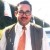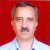# Distribution of Customer Satisfaction Survey

Six Sigma – iSixSigma Forums General Forums Methodology Distribution of Customer Satisfaction Survey

Viewing 5 posts - 1 through 5 (of 5 total)
• Author
Posts
• #54150Neeraj
Participant

Which statistical distribution a customer satisfaction survey follows ? Can one treat it normally distributed? If yes, is it possible to calculate Z score of this data using LSL = 0 and USL = 100 ?

0
#193939manipage
Guest

Hi Neeraj,

I am not sure, in what format your data is, but if you have measured customer satisfaction in terms of percentage i.e. 50% 70% etc satisfied, you can set a percentage below which the data will be defective, can try to do the normality test with the data or can treat data as discrete data and finding OFE can calculate DPMO and then subsequently calculate your Z value.

0
#193953Usman Aziz
Member

@neerajyadav If for example your respondents are rating each question on a 1 to 10 scale (my preferred scale where 1 is not at all satisfied and 10 is extremely or completely satisfiedbut you can use different scales) and you define a defect as any rating below 7 (respondent was unsatisfied), then yes you can treat the data as normally distributed in order to calculate the z score (Sigma score) as I will explain below.

Lets say you have 100 respondents (your sample size) and according to your defect criteria 70 of them or 70% were satisfied (scored 7 or greater), then this is the %yield you would use to determine the corresponding 1-tailed z-score (%yield is simply the reverse of the %defect and = 1 – % defect). This can easily be done in MS Excel for a process yield with the formula =normsinv()+1.5 for the Short Term z score as used for many Sigma score look-up tables (with the 1.5 standard deviation shiftdebates on the shift are rifejust accept it for now :-)). In this example the formula for normsinv(0.7)+1.5 gives a value of 2.02 for your z score.

By the way, I find calculating a z score less useful for a survey than calculating the precision, that is the % margin of error in your result. Stakeholders are fine with 70% of respondents were satisfied and stating this as a z score doesnt provide much additional value (unless you get to the upper 90% range).

However stating that 70% of respondents were satisfied with a sample margin of error of +/- 9% as in the % of the population satisfied may be between 61% and 79% at a 95% confidence level is more useful in my experience.

The margin of error at 95% confidence = 1.96xsqrt(p(1-p)/n) where p is the proportion yield expected or observed, and n is your sample size. (If you havent collected any data yet, the convention is to set p=0.5 to estimate what your margin of error for a given sample size will be0.5 gives the maximum margin of error and is thus a conservative figureyou can recalculate your margin of error once you collect your actual sample.)

In this example I calculated the margin of error as follows: 1.96xsqrt(0.7×0.3/100) = 1.96xsqrt(0.21/100) = 1.96xsqrt(0.0021) = 1.96 x 0.0458 = 0.09 or 9%. Note that if the sample size n were greater than 5% of the population N, you would multiply this 9% by a Finite Population Correction factor of sqrt((N-n)/(N-1)) to reflect greater precision. In fact you can always multiply by this factor as its affect on the final margin of error is insignificant when n/N drops to 5% or less. Go ahead and try it out in Excel to get a feel for it.

0
#193959Usman Aziz
Member

@neerajyadav Thank you for the nice comments and good questions. Im not a statistician but these are things Ive been taught/learned and Im happy if they help anyone.

About using normsinv its really the same as what @Manipage said. In general there are two ways to calculate your z scorea continuous method which requires normal data, and a discrete method which simply requires the determination of the process yield. Sure continuous data is better whenever you can use it, but the discrete method is a good fallback. For satisfaction surveys with a % satisfied and where scales are rarely continuous and responses are typically skewed the discrete method is a great fit.

I asked the same question you are asking many years ago when first using the normsinv functionif I cant use the continuous method due to non-normality, then why am I using a yield to get a z score from a standard normal distribution? Ive learned to accept it as at least a consistent approach for calculating a process z score for attribute data. (If someone were to explain it again to me now in a way I could understand that would be great, but in the meantime I can still use it).

As for the 10 point scale, thats what my statistician friends at AIG R&D recommended after consulting with peers when we were collaborating on some surveys. Its far from universally accepted but has the following benefits:
 It has 7 or more points in an interval arrangement which allows us to treat the data in a semi-continuous manner (e.g. calculate mean/median and use continuous/parametric statistical tools with caution)
 The scale has no center forcing a respondent to select on one side or the other
 People are used to rating on a scale of 1 to 10 so it is more natural (easier to capture over the phone as wellonly two anchor points one at each endnot at all, and extremely)
 Biggest benefit is that you have 5 points of discrimination for lack of satisfaction and 5 points of discrimination for satisfactionthis provides a good baseline to detect changes in performance

However 5-7 point Likert and other scales have their placeit depends on the purpose of the question. Capturing continuous data where feasible is great too. The trick is to maintain as much consistency of scale as feasible while picking the right scale/question wording to get you the information you need from the respondent reliably (testing your survey questions is a great way to help validate this). This is as much an art as it is a science and a huge topic on its own. With regard to your scale, respondents may find it more awkward to try and estimate their percent satisfaction than simply expressing their level of satisfaction on an increasing scale, or on a Likert scale.

My off-hand comment about not seeing much value in calculating a z score unless you have high yield is simply based on my experience that it is more trouble trying to explain to stakeholders the concept of a z score than simply using the % yield. However when you get processes with yields like 98% (e.g. network availability), it becomes more useful as instead of being 2% shy of 100%, you are at 3.55 Sigma when you could be at 6 so lots of room to improve (provided the cost of poor quality or business case supported the need ;-)). In an organization with more mature exposure to Six Sigma, by all means its better to calculate the z score which among other benefits better reflects that its easier to make large changes in % yield at lower performance levels, whereas small changes in yield at higher performance levels move the z score needle more.

0
#193962Anand Chitanand
Participant

Excellent explanation Usman Aziz. It was extremely helpful.
Thanks ….. anand

0
Viewing 5 posts - 1 through 5 (of 5 total)

You must be logged in to reply to this topic.